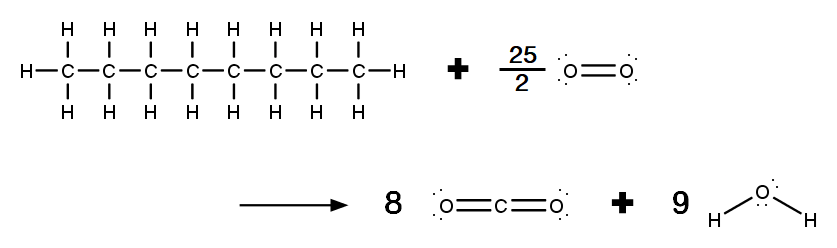# Problem: C8H18(l)+252O2(g)→8CO2(g)+9H2O(g)Part ACalculate ΔHrxn for the combustion of octane (C8H18), a component of gasoline, by using average bond energies. Express your answer using three significant figures.ΔHrxn = _____ kJ/molPart BCalculate ΔHrxn for the combustion of octane by using enthalpies of formation from Appendix IIB in the textbook. The standard enthalpy of formation of C8H18 is -250 kJ/mol.Express your answer using three significant figures.ΔHrxn = _____ kJ/molPart CWhat is the percent difference between the two results? Express your answer using two significant figures. _____ %Part DWhich result would you expect to be more accurate?a) the value calculated from the heats of formation.b) the value calculated from the average bond energies.

###### FREE Expert Solution

Given reaction: C8H18(l) + 25/2 O2(g) → 8 CO2(g) + 9 H2O(g)

Part A:

Lewis Structures of the reaction:Recall:

Average Bond Energies: found online or in textbooks

Reactants/Broken:                        Products/Formed:

• C–H = 410 kJ/mol                       • C=O = 732 kJ/mol

• C–C = 350 kJ/mol                       • O–H = 460 kJ/mol

• O=O = 498 kJ/mol

91% (468 ratings)###### Problem Details

C8H18(l)+252O2(g)→8CO2(g)+9H2O(g)

Part A

Calculate ΔHrxn for the combustion of octane (C8H18), a component of gasoline, by using average bond energies. Express your answer using three significant figures.

ΔHrxn = _____ kJ/mol

Part B

Calculate ΔHrxn for the combustion of octane by using enthalpies of formation from Appendix IIB in the textbook. The standard enthalpy of formation of C8H18 is -250 kJ/mol.Express your answer using three significant figures.

ΔHrxn = _____ kJ/mol

Part C

What is the percent difference between the two results? Express your answer using two significant figures.

_____ %

Part D

Which result would you expect to be more accurate?

a) the value calculated from the heats of formation.

b) the value calculated from the average bond energies.